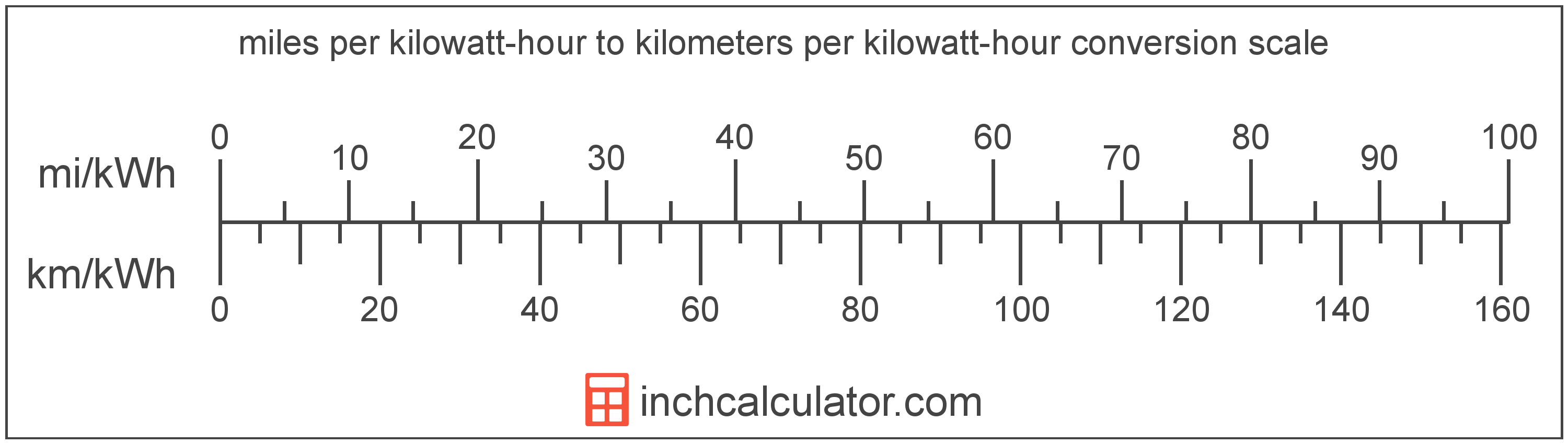# Kilometers per Kilowatt-Hour to Miles per Kilowatt-Hour Converter (Km/kWh to Mi/kWh)

Enter the electric car efficiency in kilometers per kilowatt-hour below to get the value converted to miles per kilowatt-hour.

Results in Miles per Kilowatt-Hour:1 km/kWh = 0.621371 mi/kWh

Do you want to convert mi/kWh to km/kWh?

## How to Convert Kilometers per Kilowatt-Hour to Miles per Kilowatt-Hour

Convert kilometers per kilowatt-hour to miles per kilowatt-hour with this simple formula:

mi/kWh = km/kWh ÷ 1.609344

Insert the km/kWh efficiency measurement in the formula and then solve to find the result.

For example, let's convert 50 km/kWh to mi/kWh:

50 km/kWh = ( 50 ÷ 1.609344 ) = 31.06856 mi/kWhKilometers per kilowatt-hour and miles per kilowatt-hour are both units used to measure electric car efficiency. Keep reading to learn more about each unit of measure.

## What Are Kilometers per Kilowatt-Hour?

Kilometers per kilowatt-hour is a measure of electric vehicle energy efficiency equivalent to the number of kilometers a vehicle can travel using one kilowatt-hour of energy.

The kilometer per kilowatt-hour is an SI unit of electric car efficiency in the metric system. Kilometers per kilowatt-hour can be abbreviated as km/kWh; for example, 1 kilometer per kilowatt-hour can be written as 1 km/kWh.

In the expressions of units, the slash, or solidus (/), is used to express a change in one or more units relative to a change in one or more other units.

## What Are Miles per Kilowatt-Hour?

Miles per kilowatt-hour is a measure of electric vehicle efficiency equal to the distance traveled in miles consuming one kilowatt-hour of energy.

The mile per kilowatt-hour is a US customary unit of electric car efficiency. Miles per kilowatt-hour can be abbreviated as mi/kWh; for example, 1 mile per kilowatt-hour can be written as 1 mi/kWh.

## Kilometer per Kilowatt-Hour to Mile per Kilowatt-Hour Conversion Table

Table showing various kilometer per kilowatt-hour measurements converted to miles per kilowatt-hour.
Kilometers Per Kilowatt-hour Miles Per Kilowatt-hour
1 km/kWh 0.621371 mi/kWh
2 km/kWh 1.2427 mi/kWh
3 km/kWh 1.8641 mi/kWh
4 km/kWh 2.4855 mi/kWh
5 km/kWh 3.1069 mi/kWh
6 km/kWh 3.7282 mi/kWh
7 km/kWh 4.3496 mi/kWh
8 km/kWh 4.971 mi/kWh
9 km/kWh 5.5923 mi/kWh
10 km/kWh 6.2137 mi/kWh
11 km/kWh 6.8351 mi/kWh
12 km/kWh 7.4565 mi/kWh
13 km/kWh 8.0778 mi/kWh
14 km/kWh 8.6992 mi/kWh
15 km/kWh 9.3206 mi/kWh
16 km/kWh 9.9419 mi/kWh
17 km/kWh 10.56 mi/kWh
18 km/kWh 11.18 mi/kWh
19 km/kWh 11.81 mi/kWh
20 km/kWh 12.43 mi/kWh
21 km/kWh 13.05 mi/kWh
22 km/kWh 13.67 mi/kWh
23 km/kWh 14.29 mi/kWh
24 km/kWh 14.91 mi/kWh
25 km/kWh 15.53 mi/kWh
26 km/kWh 16.16 mi/kWh
27 km/kWh 16.78 mi/kWh
28 km/kWh 17.4 mi/kWh
29 km/kWh 18.02 mi/kWh
30 km/kWh 18.64 mi/kWh
31 km/kWh 19.26 mi/kWh
32 km/kWh 19.88 mi/kWh
33 km/kWh 20.51 mi/kWh
34 km/kWh 21.13 mi/kWh
35 km/kWh 21.75 mi/kWh
36 km/kWh 22.37 mi/kWh
37 km/kWh 22.99 mi/kWh
38 km/kWh 23.61 mi/kWh
39 km/kWh 24.23 mi/kWh
40 km/kWh 24.85 mi/kWh